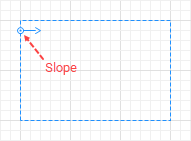AnyLogic

# Sloped nodes

In Rectangular node and Polygonal node you can define a slope that will be applied for pedestrian movement only. This is needed when you simulate stairs connecting two floors, see the demo model below.

Demo model: Stairs

You define a slope for the area using the special markup element named Slope.

Demo model: Sloped Area

To define a slope in the node

1. To make the node sloped, first select the Sloped option in the node’s Position and size properties.
2. The slope line will appear inside the node in the graphical editor:3. In the graphical editor, adjust the slope line’s direction. The line should point exactly in the Z-coordinates growth direction.
4. Click the slope line and tune it to define the required slope.
5. If you know the angle value, leave Define slope as: angle option selected and specify the angle value in the Vertical angle control below.
6. Otherwise, if you know not the angle, but know the height between the levels, choose guiding line in the slope line’s Define slope as property. In the dZ property below, enter the height between the levels, in pixels. Then direct the slope line in the graphical editor so that in XY plane it lies parallel to the escalator or stairs area direction.

## Slope properties

General

Define slope as — Choose here, whether you want to define a slope just by specifying the angle value, or with a guiding line, if you do not know the area slope angle, but know the area height.

Vertical angle — [Enabled if Define slope as: angle] The slope angle.

dZ — [Enabled if Define slope as: guiding line] The height between the levels, in pixels.

Position and size

X — X-coordinate of the line’s start point.

Y — Y-coordinate of the line’s start point.

Horizontal orientation — [Enabled if Define slope as: angle] Line’s rotation angle in XY plane.

dX — [Enabled if Define slope as: guiding line] X-offset of the end point regarding the start point of the line.

dY — [Enabled if Define slope as: guiding line] Y-offset of the end point regarding the start point of the line.